# Explore and visualize the parser events and performance. Pandas. Seaborn. KLib. ADTKFirst, i was going to start writing an article about researching Plotly community and changed my mind after a short while in favor of exploring how a multithreaded parser that i've implemented discourse-parser for the purpose of whether getting the community data runs performant. Did it have performance issues? Is a way of data requesting and writing worked as expected due to multithreading? Was parsing completed correctly and had no anomalies?

While the parser was working it produced a lot of logs, each log record is a specific type of event representing activity a thread is doing at a moment, for example `data.get` to request a community website. Those log records are the target to explore, visualize, and get insights from. Also, they are time series.

For a broad view on the concerns the article is split into the following sections:

• Logs Overview.
• Continuous Running Index.
• Events frequency.
• Network Latency Time Series.
• Write on Disk Time Series.

## Logs Overview and Sample

The logs file parser-latest-log.tar.bz2 that is produced by the parser to uncompress and read. Each log record inside is nothing than a json line generated by the structlog logging library. I like using that lib for events tracking. Let's print a sample of the records.

``````# Dataset
import tarfile
import json
import pandas as pd

with tarfile.open('files/parser-latest-log.tar.bz2') as tfile:
file = tfile.extractfile('parser-latest-log.txt')

json_lines = [json.loads(line) for line in logs_data.splitlines()]
df = pd.DataFrame.from_records(json_lines)
df['timestamp'] = pd.to_datetime(df['timestamp'])
``````
``````# Table
df.sample(5).to_markdown(index=False)
``````
event timestamp thread_name target args path exc_info
data.get 2023-03-12 11:55:59.029086+00:00 Consumer-11 topic {'page': '68', 'id': 67797} nan nan
data.get 2023-03-12 12:23:21.008310+00:00 Consumer-6 topic {'page': '232', 'id': 52642} nan nan
data.saved 2023-03-12 12:59:10.965976+00:00 Consumer-12 topic {'page': '447', 'id': 34298} latest/order-created/ascending-False/page-447/topic-34298-2023-03-12T11:44:38+00:00.json nan
data.saved 2023-03-12 11:59:56.268552+00:00 Consumer-2 topic {'page': '91', 'id': 65603} latest/order-created/ascending-False/page-91/topic-65603-2023-03-12T11:44:38+00:00.json nan
task.get 2023-03-12 11:46:53.948818+00:00 Producer-2 latest nan nan nan

The handy library klib brings a brief dataset overview visualizing categories(a few column in the sample table), the most and least frequent value counts, values density per category. There are three categories we need to plot `event`, `thread_name`, `target`. The others `args`, `path`, `exc_info` are for the debugging purpose, `timestamp` is considered in other sections.

``````import matplotlib.pyplot as plt
import klib

plt.suptitle('')
``````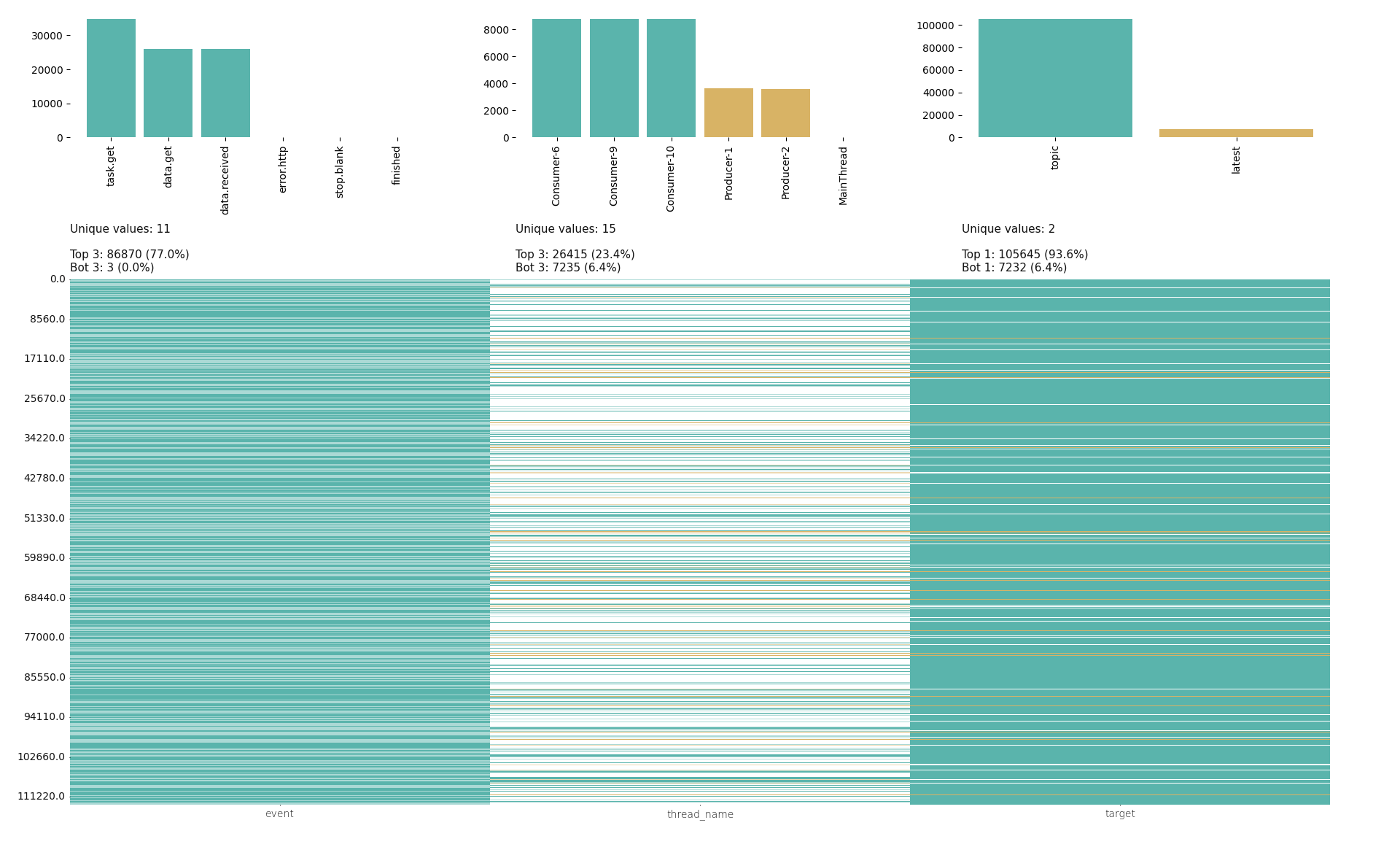As mentioned, there are two bloks representing each column, frequency and density. Density is a bottom block filled in with the thin lines, those lines are the most and least frequent values in the upper block. Zoom in the image to see text details.

A few interesting facts from the image:

• The `event`'s most filled the vertical bar with teal color almost full `77.0%`
• While the `thread_name`'s most are not so frequent within all the category values, its vertical bar contains a lot of white color that means filling in with other values.
• In the `target` bar only two unique values and the most frequent value `topic` filled the bar in for `93.6%`

## Continuous Running Index

The index is simply a number that is expected to be constant, doesn't matter what a value is. Constant means there were no interruptions of parser running. The basic way to visualize such the number is just a line, straight line. So, Is that line straight?

``````# Dataset

.pivot(columns='event', values='event')\
.resample('min')\
.count()\

ts_diff_df = events_per_min_df.reset_index()\
.diff()\
.loc[:, 'timestamp']
ts_diff_df = ts_diff_df.dt.seconds / 60  # Convert to minutes
ts_diff_df.rename('Diff in minutes', inplace=True)
``````
``````# Plot
import seaborn as sns

sns.set_theme(style='white', context={'axes.labelsize': 16, 'axes.titlesize': 16, 'legend.fontsize': 16})
sns.set_palette('pastel')
sns.set_style({'axes.spines.left': False, 'axes.spines.bottom': False, 'axes.spines.right': False, 'axes.spines.top': False})

line = sns.lineplot(data=ts_diff_df, lw=4)
last_pos = line.get_xticks()[-2]
line.set_xticks([1, last_pos],
labels=[events_per_min_df.index.min().strftime('%H:%M:%S'),
events_per_min_df.index.max().strftime('%H:%M:%S')])
line.figure.suptitle('Continuous Index', size=20)
``````Throughout all the running time`Timedelta('0 days 02:20:00')` there were no interruptions as the straight line of constant value `1` means that difference between every consequent ticks is 1 min, no more no less.

## Events Frequency

`event` is the most valuable category in a parser work. We can manually count and interpret logs for how many each event type was produced, whom, when, etc. That way works fine if log records are a few, that's not our case. Records are thousands, print the dataset shape `df.shape` is `112880`.

### Frequency Per Target

To make a general picture about all the events we can group them up into 5 minutes bins(groups) and split per `target` to visualize the dataset in different dimensions at the same time. Grouping into small time ranges(bins) with event frequency(count) calculation is being made for having data visualization easy to interpret. So, we will see how often each `event` type is produced per `target` within `5 min` bins.

Only the events `task.get`, `data.get`, `data.received`, `data.saved` are left as the rest is about technical tracking and errors. Errors should be considered separately, i've manually checked their count and they are a couple.

``````# Datset
sub_df = df.drop(columns=['args', 'path', 'exc_info'])\
.dropna(subset=['target'])\
.sort_index(axis=1)

events_occured_df = sub_df.pivot(index='timestamp',
values='event')\
.fillna(0)\
.melt(ignore_index=False)\
.query('value == 1')\
.drop(columns='value')\
.sort_index()

cut_bins = pd.date_range(start=events_occured_df.index.min() , end=events_occured_df.index.max() + pd.Timedelta(minutes=5), freq='5min')
cut_labels = list(f'{i}th' for i in range(5, len(cut_bins) * 5, 5))

events_reduced_df = events_occured_df[~events_occured_df['event'].isin(['do', 'done', 'stop.blank', 'error.http'])]
events_reduced_df.loc[:, '5min bin'] = pd.cut(events_reduced_df.index,
bins=cut_bins,
labels=cut_labels,
include_lowest=True)
``````
``````# Plot
displot = sns.displot(data=events_reduced_df,
x='5min bin',
hue='event',
col='target',
row='event',
multiple='stack')
loc, labels = plt.xticks()
displot.set_xticklabels(labels, rotation=45)
displot.fig.suptitle('Events Frequency Over Targets', size=20)
``````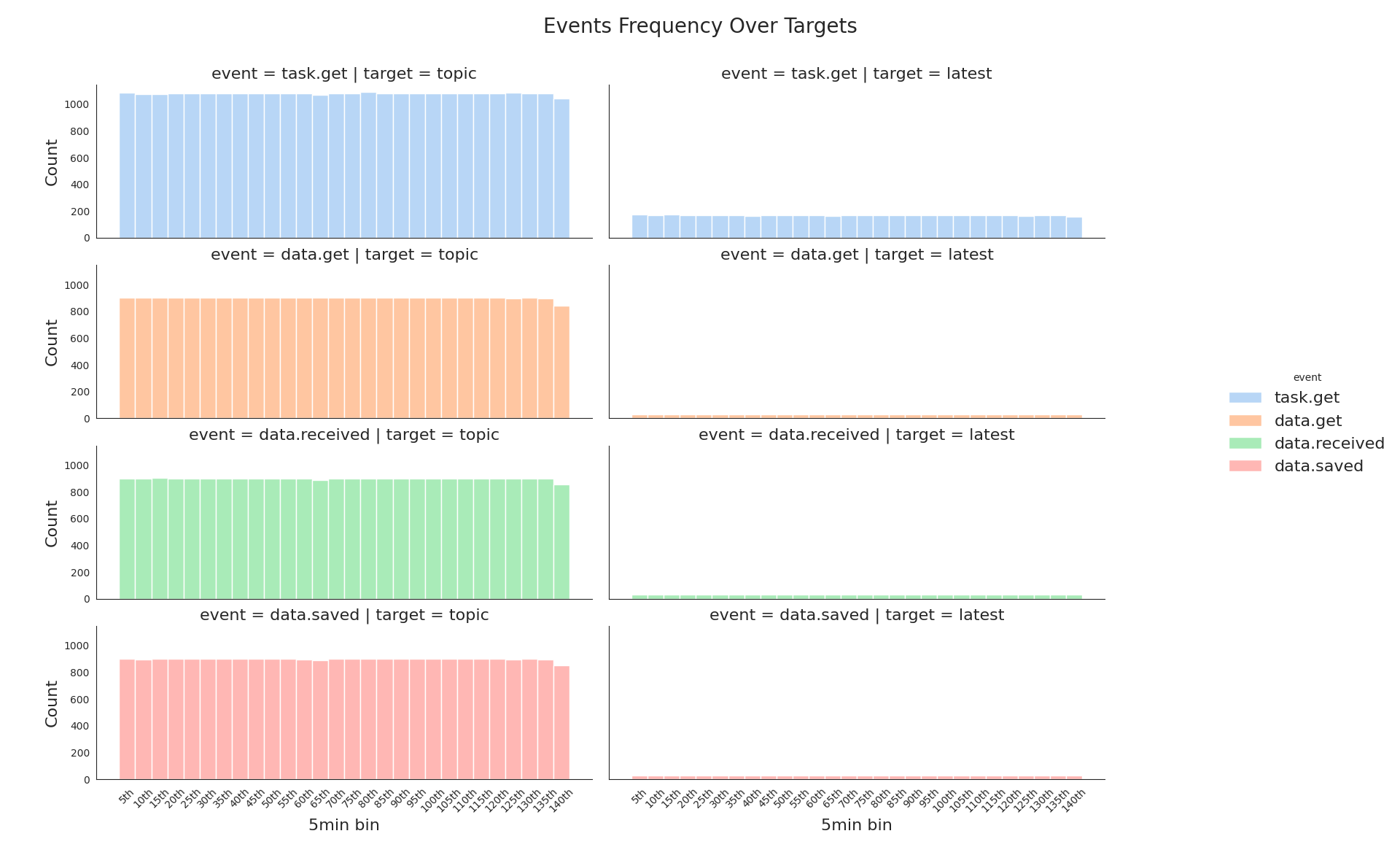Nice, right away we see:

• That event's amount of the target `latest` is an order smaller than of `topic`.
• The event `task.get` amount is bigger at both targets than other event types, but it's not representative of the plot type. It can be depicted better.

Visualizing the event amount as a ratio could help with the feeling of how much bigger `task.get` is.

``````with sns.axes_style('whitegrid'):
displot = sns.displot(data=events_reduced_df,
x=events_reduced_df['5min bin'].apply(lambda val: val.removesuffix('th'))\
.astype(int)\
.rename('5min int bin'),
hue='event',
col='target',
multiple='fill',
kind='kde')
displot.fig.suptitle('Events Frequency Ratio', size=20)
``````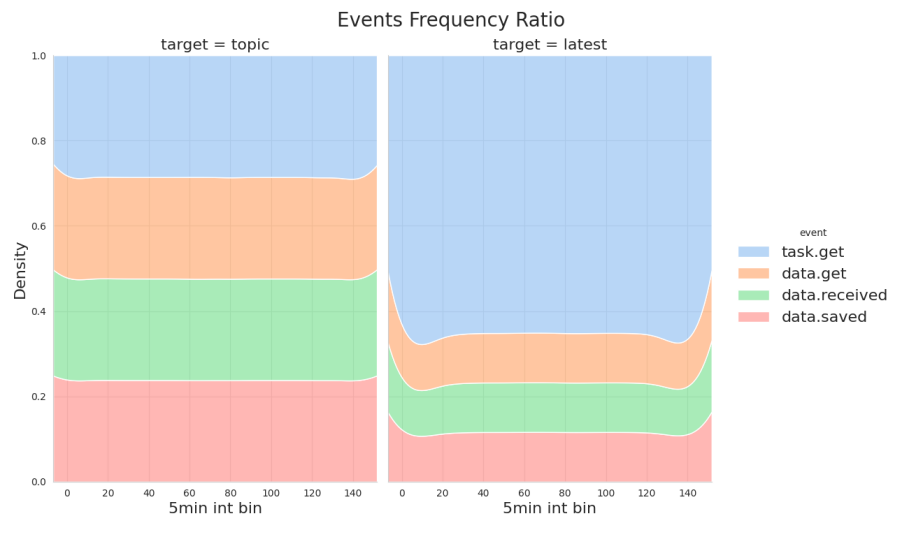Much more clear now:

• `task.get` of the target `topic` is around `1.0 - 0.7`, `0.3` of the whole. It looks balanced.
• While `task.get` of the target `latest` is around `1.0 - 0.35`, `0.65` is quite big.

A similar way to the frequency above the dataset is plotted, but a per thread dimension. How often each `event` type is produced per `thread_name` within `5 min` bins.

``````displot = sns.displot(data=events_reduced_df, x='5min bin', hue='event', col='thread_name', col_wrap=2, multiple='stack')
loc, labels = plt.xticks()
displot.set_xticklabels(labels, rotation=45)
``````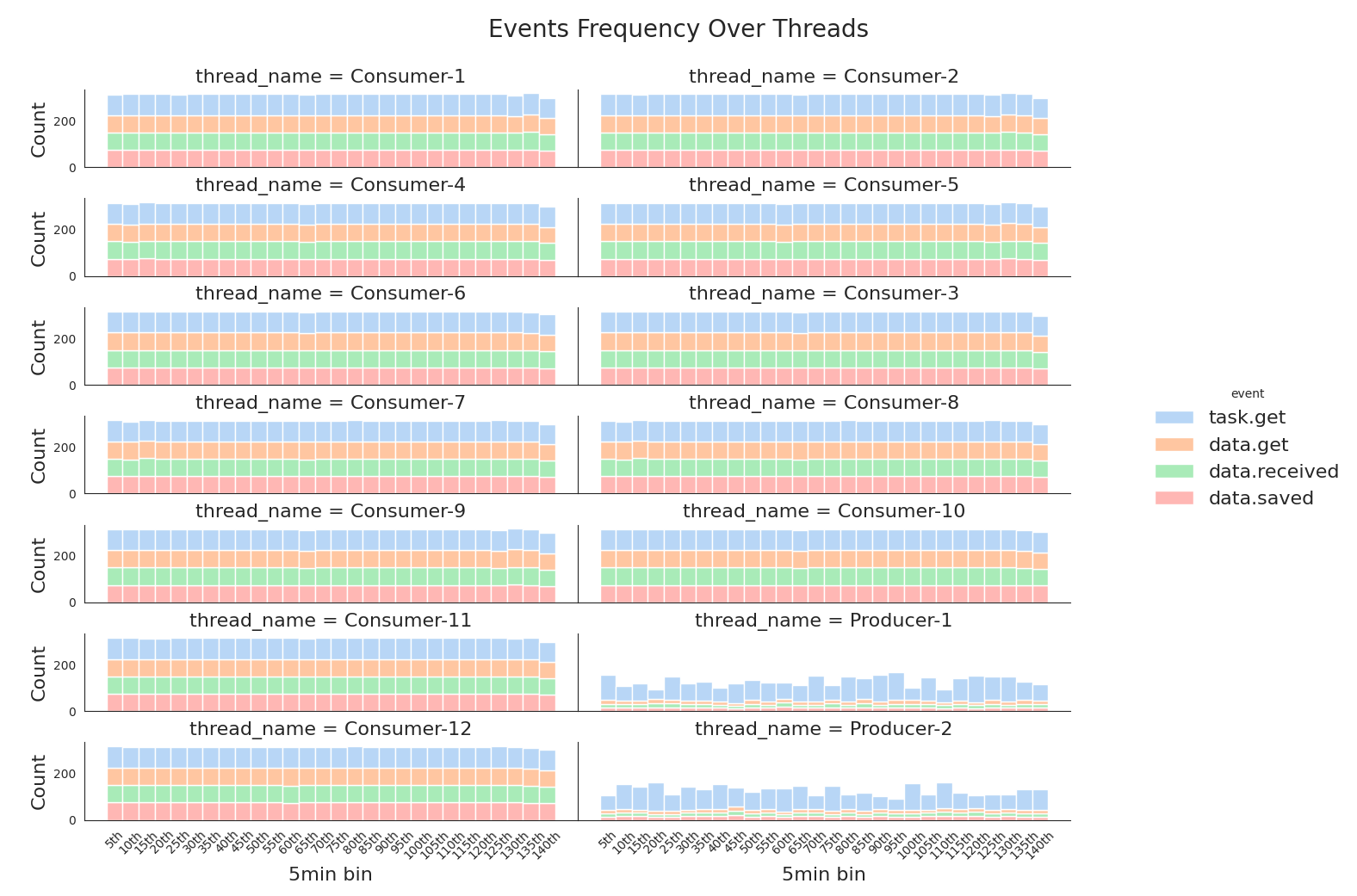The picture shows that each thread workload looks the same. That's a good observation, it means the parser was working as expected.

## Network Latency Time Series

The network latency is a time delta between the events `data.received, data.get`. In order to compute all the deltas, the dataset has to be sorted by two columns `timestamp, thread_name` to avoid misplacing when, for example, event `data.get` is followed by `data.get` but of a different thread.

The valid event order:

1. `task.get`
2. `data.get`
3. `data.received`
4. `data.saved`

### Check the events order validity

To check that an order after sorting is valid two columns should be added, the previous and next event. After columns are added, we can match accordingly to valid event order above each event with its previous and next to state it is valid in according to.

``````# Dataset
order_state_df = events_reduced_df.reset_index()\
.assign(prev_event=lambda df: df['event'].shift(1),
next_event=lambda df: df['event'].shift(-1))
``````
``````# Table
.iloc[10:16]\
.loc[:, ['timestamp', 'event', 'prev_event', 'next_event']]
``````
timestamp event prev_event next_event

The sample looks valid, now define a function with valid event order matching assigning results to new column `is_valid`.

``````# Dataset
def is_order_valid(series):
if series['event'] == 'data.get':
return series['prev_event'] == 'data.get'
if series['event'] == 'data.saved':
return None

order_state_df['is_valid'] = order_state_df.apply(is_order_valid, axis=1)
``````
``````# Table
order_state_df[['timestamp', 'event', 'prev_event', 'is_valid']].iloc[10:16]
``````
timestamp event prev_event is_valid

`is_valid` marks rows as a boolean flag. Now we can group the dataset with and without `is_valid` flag, just to visualize if group counts are different.

``````with sns.plotting_context({'ytick.labelsize': 16, 'xtick.labelsize': 0, 'xtick.major.width': 0,  'axes.labelsize': 0}):
fig, axes = plt.subplots(2, 1, sharey=False)
event_counts = order_state_df['event'].value_counts(dropna=False)\
.reset_index()
barplot = sns.barplot(data=event_counts,
ax=axes,
y='event',
x='count',
color=sns.colors.xkcd_rgb['light blue'])
axes.title.set_text('Unvalidated Event Counts')
for i in barplot.containers:
barplot.bar_label(i, fontsize=16)

valid_event_counts = order_state_df[['event', 'is_valid']]\
.value_counts(dropna=False)\
.reset_index()\
.assign(group=lambda df: df['event'].astype(str) + ' - ' + df['is_valid'].astype(str))
barplot = sns.barplot(data=valid_event_counts,
ax=axes,
y='group',
x='count',
color=sns.colors.xkcd_rgb['light blue'])
axes.title.set_text('Validated Event Counts')
for i in barplot.containers:
barplot.bar_label(i, fontsize=16)
``````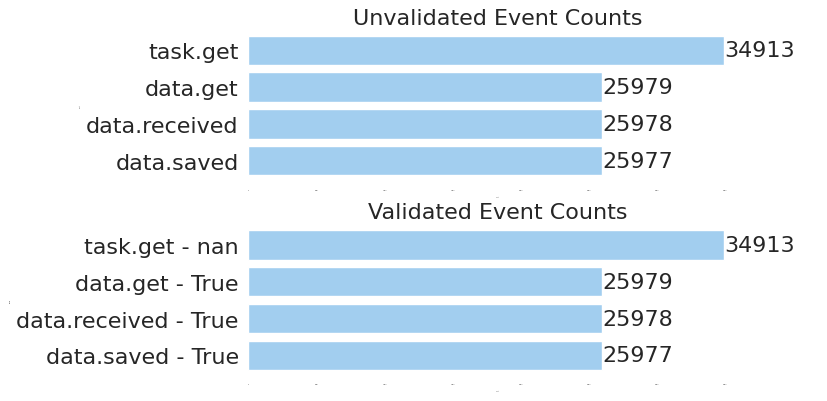Obviously, all the events are valid due to all the counts on the top and bottom charts are equal. Consequently, network latency computing can be done with no data filtering or cleaning. The whole dataset completely match valid event order, further computed time deltas are correct.

### Latency Overall

Subtract the `data.get` timestamp from `data.received` one. For making it, a new column `prev_event_timestamp` is added that contains timestamps of `data.get`. Latency are seconds.

``````# Dataset
latency_diffs = order_state_df.assign(prev_event_timestamp=lambda df: df['timestamp'].shift(1))\
.loc[``````